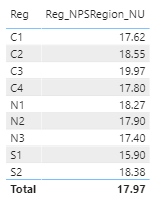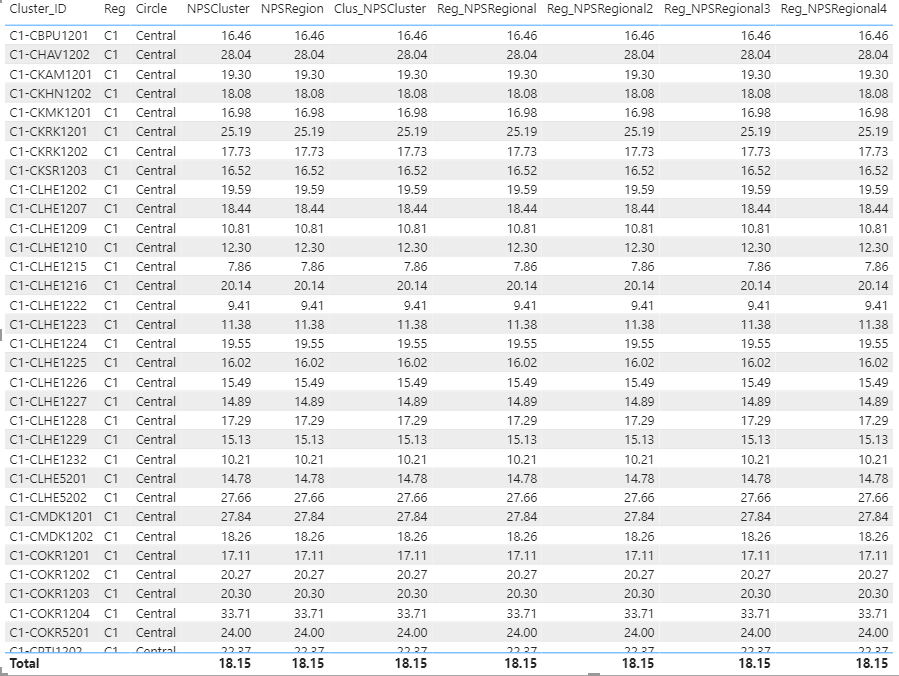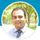cancel
Showing results for
Did you mean:Frequent Visitor

## New Table Creation Summarize Average of Average Problem & Measure Filters Not Working in New Table

I am relatively newbie in world of DAX, and stuck for almost a week on a problem. Would really appreciate your help on this.

Background:

I have a telecom network data for NPS in "nps" table. Data is at Site, Cluster and Region levels.

Mapping is like:

Site -> Cluster -> Reg -> Network

To calculate Network NPS I defined measure:

NPSNetwork = CALCULATE( 'nps'[NPS], REMOVEFILTERS() )

To calculate Region NPS I defined measure:

NPSRegion = CALCULATE( 'nps'[NPS], ALLEXCEPT('nps', 'nps'[Reg]) )

where NPS is another calculated measure:
NPS =
var denom = COUNT( 'nps'[Customer] )
return
DIVIDE(
100*(
CALCULATE( COUNT( 'nps'[Customer] ), 'nps'[Customer] = "Promoter" ) -
CALCULATE( COUNT( 'nps'[Customer] ), 'nps'[Customer] = "Detractor" )
), denom)

My problem is that when in same table I generate visual on region, the Total i.e. Network value from NPSRegion measure is correct 18.15:Question 1:

But then I created a calculated table at Region level from "nps" table and used average of "NPS" measure, but the resultant Total value is not correct. It yields 17.97 instead of 18.15. That value is the average of resultant 9 values, what we commonly refer to as average of average problem. How this can be solved? I solved it using another measure in the new table referring to columns of nps table, that I don't think is an effective approach.

New regional level table query:

NPS_Region = SUMMARIZECOLUMNS( nps[Reg], "Reg_NPSRegion_NU", nps[NPSRegion] )I had a work around to define another measure in the new table referring to columns of old table:
Reg_NPSRegional = CALCULATE( 'nps'[NPS], ALLEXCEPT('NPS_Region', 'NPS_Region'[Reg]) )

Question 2:
I similarly created another table at Cluster Level and now I want to put regional NPS to compare every cluster value to it's regional value, but the regional NPS measure does not give correct value with Cluster Filter. I tried experimenting with filters, calculate, AllExcept but to no avail. I just couldn't make it work:Reg_NPSRegional2 =
IF(
HASONEVALUE( 'nps'[Reg] ) || HASONEVALUE( NPS_Region[Reg] ) || HASONEVALUE( Cluster_Region_Mapping[Reg] ) || HASONEVALUE( Region_Circle_Mapping[Reg] ),
'nps'[NPS],
CALCULATE( 'nps'[NPS], ALL('nps') )
)

Reg_NPSRegional3 =
IF(
HASONEFILTER( 'nps'[Reg] ) || HASONEFILTER( NPS_Region[Reg] ) || HASONEFILTER( Cluster_Region_Mapping[Reg] ) || HASONEFILTER( Region_Circle_Mapping[Reg] ),
'nps'[NPS],
CALCULATE( 'nps'[NPS], ALL('nps') )
)

3 REPLIES 3Anonymous
Not applicable
I don't have time right now to deal with this but I can give you some advice. First, measures should never be preceded by the name of the table they belong to. NEVER. They must stand on their own. Second, using ALLEXCEPT without a deep understanding of what it does in different contexts is a very good and fast way to get wrong figures.Community Champion

Hi @ghamiz ,

Can you share sample data and expected output.

Regards,
Harsh Nathani
Did I answer your question? Mark my post as a solution! Appreciate with a Kudos!! (Click the Thumbs Up Button)Frequent Visitor

Hi, expected output of Question 1 is already shared. See second image Total Row, compare that to first image Total Row For Question 2, expected output is: